# LSTM对股票的收益进行预测（Keras实现）

1、数据特征归一化（标准化）

2、将数据集转化为有监督学习问题

1、搭建LSTM模型并绘制损失图

2、预测并反转数据（反归一化）

3、绘制模型预测结果图

## 一、概述：

import pandas as pd
# 核心代码，设置显示的最大列、宽等参数，消掉打印不完全中间的省略号
pd.set_option('display.max_columns', 1000)
pd.set_option('display.width', 1000)
pd.set_option('display.max_colwidth', 1000)

import matplotlib.pyplot as plt
import numpy as np
from pandas import DataFrame
from pandas import concat
from sklearn.preprocessing import MinMaxScaler
from keras.models import Sequential
from keras.layers import LSTM,Dense,Dropout
from numpy import concatenate
from sklearn.metrics import mean_squared_error,mean_absolute_error,r2_score
from math import sqrt

## 二、股票数据准备

#定义字符串转换为浮点型（此处是转换换手率）
def str_to_float(s):
s=s[:-1]
s_float=float(s)
return s_float

## 读取excel文件，并将‘日期’列解析为日期时间格式,并设为索引
stock_data.drop(['交易日期','成交量','市值','pb'],axis=1, inplace=True) #删除多列数据
stock_data['换手率']=stock_data['换手率'].apply(str_to_float)
#对股票数据的列名重新命名
stock_data.columns=['open','high','low','close','turnover','pe']
stock_data.index.name='date' #日期为索引列
#将数据按日期这一列排序（保证后续计算收益率的正确性）
stock_data=stock_data.sort_values(by='date')
# 增加一列'earn_rate', 存储每日的收益率
stock_data['earn_rate'] = stock_data['close'].pct_change()
#缺失值填充
stock_data['earn_rate'].fillna(method='bfill',inplace=True)
# 打印数据的前5行
print(stock_data.head())

部分数据如下：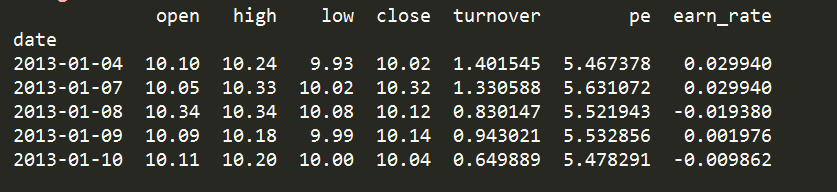## 三、股票数据预处理

### 1、数据特征归一化（标准化）

LSTM对输入数据的规模很敏感，特别是当使用sigmoid（默认）或tanh激活函数时。需要将数据重新调整到0到1的范围（也称为标准化）。本实验使用scikit-learn库中的MinMaxScaler预处理类实现数据集的规范化。

#获取DataFrame中的数据，形式为数组array形式
values=stock_data.values
#确保所有数据为float类型
values=values.astype('float32')

# 特征的归一化处理
scaler = MinMaxScaler(feature_range=(0, 1))
scaled = scaler.fit_transform(values)
print(scaled)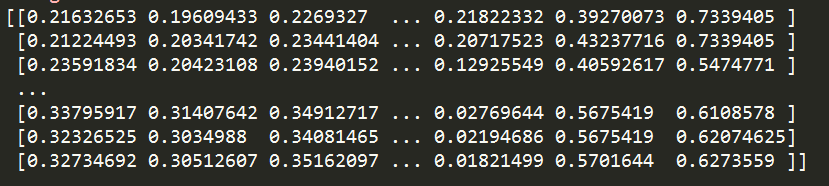### 2、将数据集转化为有监督学习问题

#定义series_to_supervised()函数
#将时间序列转换为监督学习问题
def series_to_supervised(data, n_in=1, n_out=1, dropnan=True):
"""
Frame a time series as a supervised learning dataset.
Arguments:
data: Sequence of observations as a list or NumPy array.
n_in: Number of lag observations as input (X).
n_out: Number of observations as output (y).
dropnan: Boolean whether or not to drop rows with NaN values.
Returns:
Pandas DataFrame of series framed for supervised learning.
"""
n_vars = 1 if type(data) is list else data.shape
df = DataFrame(data)
cols, names = list(), list()
# input sequence (t-n, ... t-1)
for i in range(n_in, 0, -1):
cols.append(df.shift(i))
names += [('var%d(t-%d)' % (j+1, i)) for j in range(n_vars)]
# forecast sequence (t, t+1, ... t+n)
for i in range(0, n_out):
cols.append(df.shift(-i))
if i == 0:
names += [('var%d(t)' % (j+1)) for j in range(n_vars)]
else:
names += [('var%d(t+%d)' % (j+1, i)) for j in range(n_vars)]
# put it all together
agg = concat(cols, axis=1)
agg.columns = names
# drop rows with NaN values
if dropnan:
agg.dropna(inplace=True)
return agg


• data：输入数据需要是列表或二维的NumPy数组的观察序列。
• n_in：输入的滞后观察数（X）。值可以在[1..len（data）]之间，可选的。默认为1。
• n_out：输出的观察数（y）。值可以在[0..len（data）-1]之间，可选的。默认为1。
• dropnan：Bool值，是否删除具有NaN值的行，可选的。默认为True。

• 返回：用于监督学习的Pandas DataFrame。

#将时间序列转换为监督学习问题
reframed = series_to_supervised(scaled, 1, 1)
# 删除不想预测的特征列，这里只预测收益率
reframed.drop(reframed.columns[[7,8,9,10,11,12]], axis=1, inplace=True)
# 打印数据的前5行
print(reframed.head())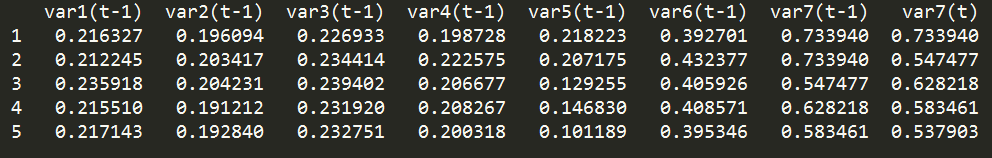## 四、股票数据划分为训练集和测试集

Keras LSTM层的工作方式是通过接收3维（N，W，F）的数字阵列，其中N是训练序列的数目，W是序列长度，F是每个序列的特征数目。

# 划分训练集和测试集
values = reframed.values
train = np.concatenate([values[:774, :],values[1219:,:]])
test = values[774:1219, :]
# 划分训练集和测试集的输入和输出
train_X, train_y = train[:, :-1], train[:, -1]
test_X, test_y = test[:, :-1], test[:, -1]
#转化为三维数据
# reshape input to be 3D [samples, timesteps, features]
train_X = train_X.reshape((train_X.shape, 1, train_X.shape))
test_X = test_X.reshape((test_X.shape, 1, test_X.shape))
print(train_X.shape, train_y.shape)
print(test_X.shape, test_y.shape)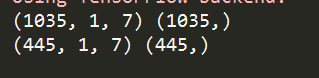## 五、模型构建及其预测

### 1、搭建LSTM模型并绘制损失图

# 搭建LSTM模型
model = Sequential()
# fit network
history = model.fit(train_X, train_y, epochs=50, batch_size=100, validation_data=(test_X, test_y), verbose=2,shuffle=False)

# 绘制损失图
plt.plot(history.history['loss'], label='train')
plt.plot(history.history['val_loss'], label='test')
plt.title('LSTM_600000.SH', fontsize='12')
plt.ylabel('loss', fontsize='10')
plt.xlabel('epoch', fontsize='10')
plt.legend()
plt.show()

完成训练和测试后绘制损失图如下：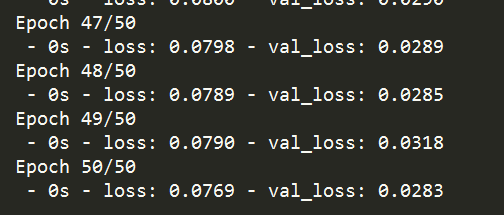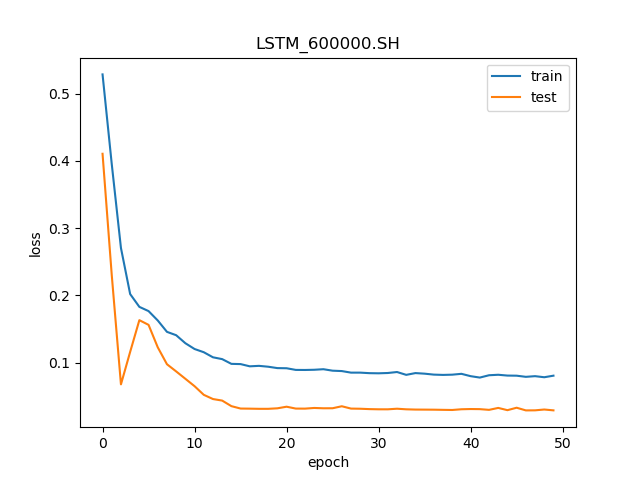### 2、预测并反转数据（反归一化）

#模型预测收益率
y_predict = model.predict(test_X)
test_X = test_X.reshape((test_X.shape, test_X.shape))

# invert scaling for forecast
#将预测结果按比例反归一化
inv_y_test = concatenate((test_X[:, :6],y_predict), axis=1)
inv_y_test = scaler.inverse_transform(inv_y_test)
inv_y_predict=inv_y_test[:,-1]

# invert scaling for actual
#将真实结果按比例反归一化
test_y = test_y.reshape((len(test_y), 1))
inv_y_train = concatenate((test_X[:, :6],test_y), axis=1)
inv_y_train = scaler.inverse_transform(inv_y_train)
inv_y = inv_y_train[:, -1]
print('反归一化后的预测结果：',inv_y_predict)
print('反归一化后的真实结果：',inv_y)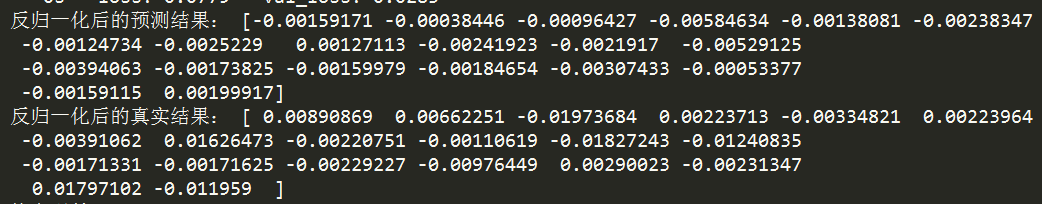### 3、绘制模型预测结果图

plt.plot(inv_y,color='red',label='Original')
plt.plot(inv_y_predict,color='green',label='Predict')
plt.xlabel('the number of test data')
plt.ylabel('earn_rate')
plt.title('2016.3—2017.12')
plt.legend()
plt.show()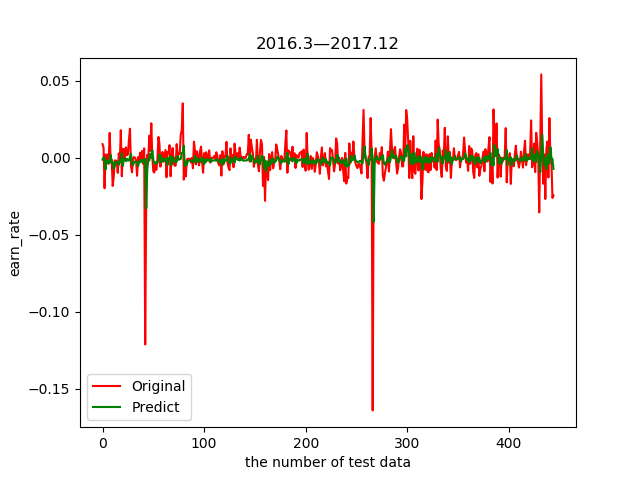## 六、模型评估


#回归评价指标
# calculate MSE 均方误差
mse=mean_squared_error(inv_y,inv_y_predict)
# calculate RMSE 均方根误差
rmse = sqrt(mean_squared_error(inv_y, inv_y_predict))
#calculate MAE 平均绝对误差
mae=mean_absolute_error(inv_y,inv_y_predict)
#calculate R square
r_square=r2_score(inv_y,inv_y_predict)
print('均方误差: %.6f' % mse)
print('均方根误差: %.6f' % rmse)
print('平均绝对误差: %.6f' % mae)
print('R_square: %.6f' % r_square)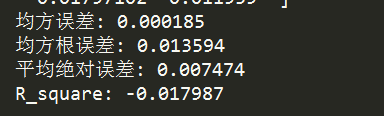3、空气污染预测

07-053万+06-284万+
01-241180
02-272133
02-1912万+
07-25523
07-301万+
01-042万+
08-222322
01-251307
01-25
04-053704
10-18608
11-17